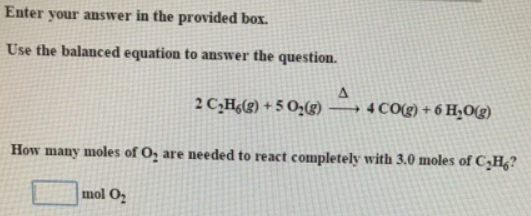# Problem: Enter your answer in the provided box.Use the balanced equation to answer the question. 2C2H6 (g) + 5O2 (g) → 4CO (g) + 6H2O (g) How many moles of O2 are needed to react completely with 3.0 moles of C 2H6?

###### FREE Expert Solution
88% (210 ratings)###### Problem Details

Use the balanced equation to answer the question.

2C2H(g) + 5O(g) → 4CO (g) + 6H2O (g)

How many moles of O2 are needed to react completely with 3.0 moles of C 2H6?